## 10. Quantum harmonic oscillator.

From (2.13), the graph of the potential energy of a one-dimensional harmonic oscillator with respect to the distance x from its equilibrium position, is a parabola with vertex at the origin O and up concave.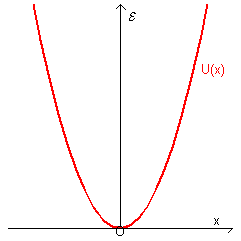A particle with finite total energy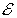that moves with harmonic motion is contained into a infinitely deep potential well so the probability to find it at infinite distance is zero.

In this case the Schrödinger equation is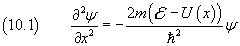and, taking U(x) from (2.13),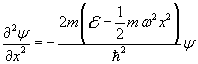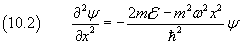This last equation can be simplified by the variable subtitution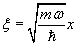from whichso, from (10.2) we have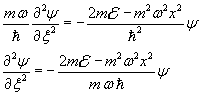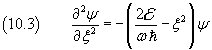We can have a more simple notation of (10.2) if we let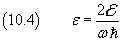so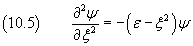The solution of this equation must approach 0 as ξ approaches positive or negative infinity: we may express it as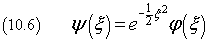where φ(ξ) is a function to be determined later.

By substituting this expression and its second derivative with respect to ξ in (10.5) we have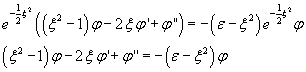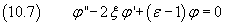Now we assume that the solution φ of (10.7) may be expressed by a series expansion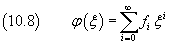and therefore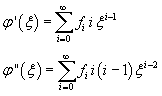By substituting these values in (10.7) we have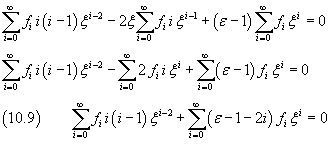Since φ does not have singularities, we cannot consider negative exponents, so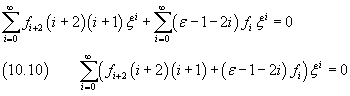The sum (10.10) is identically zero if and only if all its coefficients are zero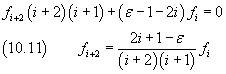The equation (10.11) establishes a recursive relationship between the coefficients of the series expansion(10.8) of φ(ξ)

Moreover, since φ(ξ) must be finite for any ξ, there must be an index n of the expansion such that the not-zero n-th term would produce the (n+2)-th term equal to 0 an so all the following terms with indexes (n+4), (n+6) and so on. Therefore, from (10.11) we obtain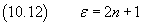that is, from (10.4)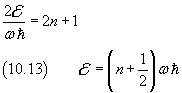If we use the Planck constant instead of the Dirac constant we have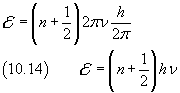The equation (10.14) represents a fundamental result in the history of Physics of the twentieth century, because it provides a theoretical justification to the Planck conjecture and improves it: harmonic oscillators, contrary to that provided by Classical Mechanics, may not have any energy, but their energy is quantized. The minimum value of the energy of a quantum oscillator id given by (10.14) with n=0. All other possible energy values differ from that for multiples of . So a harmonic oscillator can emit or absorb energy only if it exchanges with the outside blocks of energy multiples of .